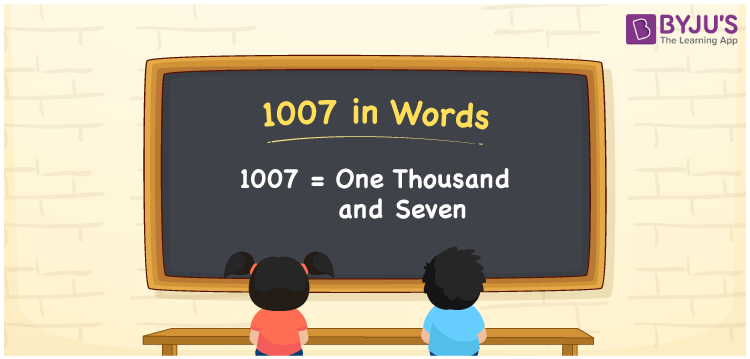# 1007 in Words

1007 in words is written as One thousand seven. In both the International System of Numerals and the Indian System of Numerals, 1007 is written as One thousand seven. The number 1007 is a Cardinal Number as it could represent some quantity.

 1007 in Words One thousand seven One thousand seven in Number 1007

## 1007 in English Words

1007 in English words is read as “One thousand seven”.## How to Write 1007 in Words?

To write 1007 in words, we shall use the place value chart. In the place value chart, put 1 in the thousands, 0 in the hundreds, 0 in the tens, and 7 in the ones, respectively. Let us make a place value chart to write the number 1007 in words.

 Thousands Hundreds Tens Ones 1 0 0 7

Thus, we can write the expanded form as

1 × Thousand + 0 × Hundred + 0 × Ten + 7 × One

= 1 × 1000 + 0 × 100 + 0 × 10 + 7 × 1

= 1000 + 0 + 0 + 7

= 1007

= One thousand seven.

1007 is a natural number, the successor of 1006 and the predecessor of 1008.

1007 in words – One thousand seven

• Is 1007 an odd number? – Yes
• Is 1007 an even number? – No
• Is 1007 a perfect square number? – No
• Is 1007 a perfect cube number? – No
• Is 1007 a prime number? – No
• Is 1007 a composite number? – Yes

## Frequently Asked Questions on 1007 in Words

Q1

### How to write 1007 in words?

1007 in words is written as One thousand seven.
Q2

### How to write 1007 in the International and Indian System of Numerals?

In both, the system of numerals, 1007 in words, is written as One thousand seven.
Q3

### How to write 1007 in a place value chart?

In the place value chart, write 1 in the thousands, 0 in the hundreds, 0 in the tens, and 7 in the ones, respectively.